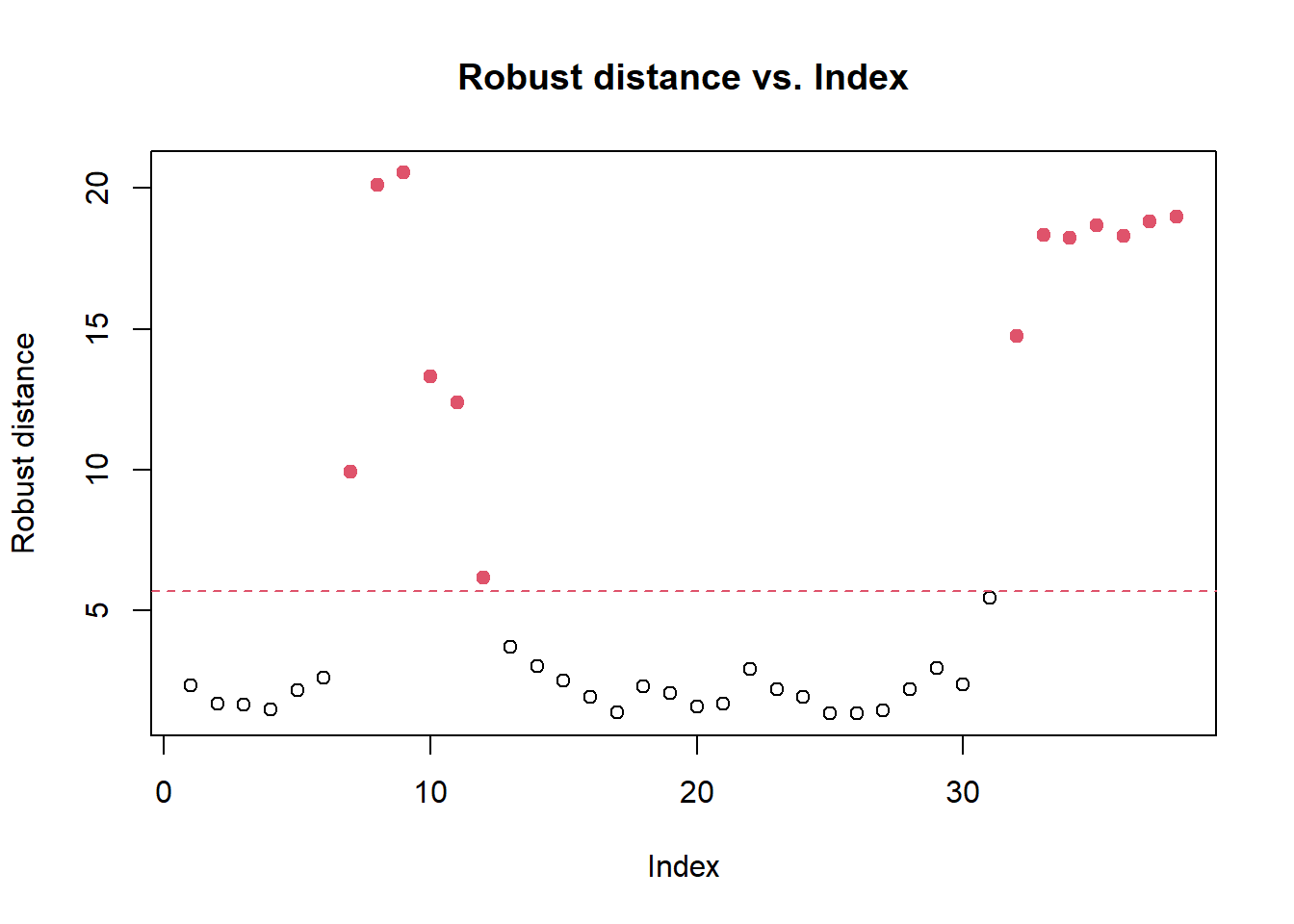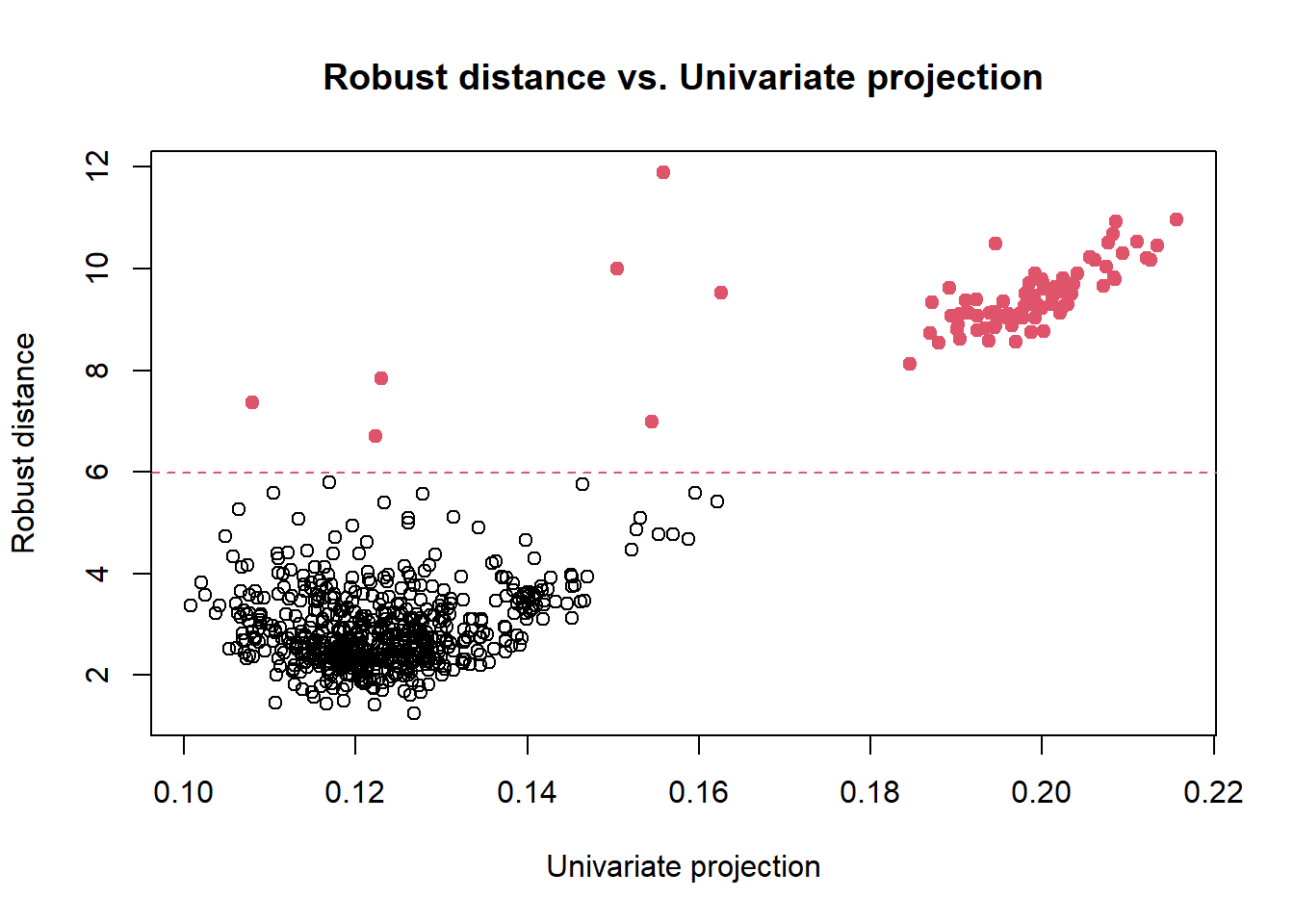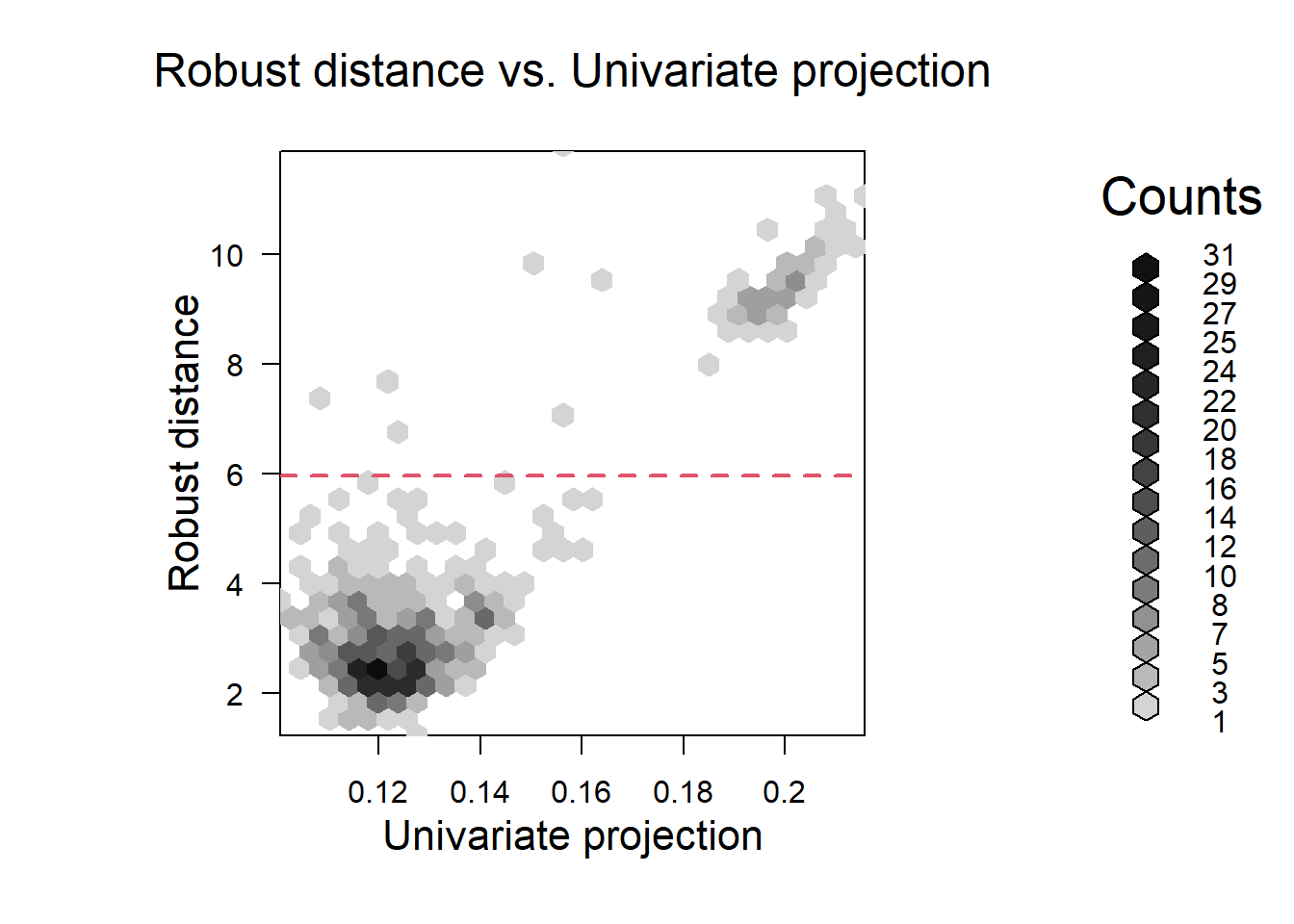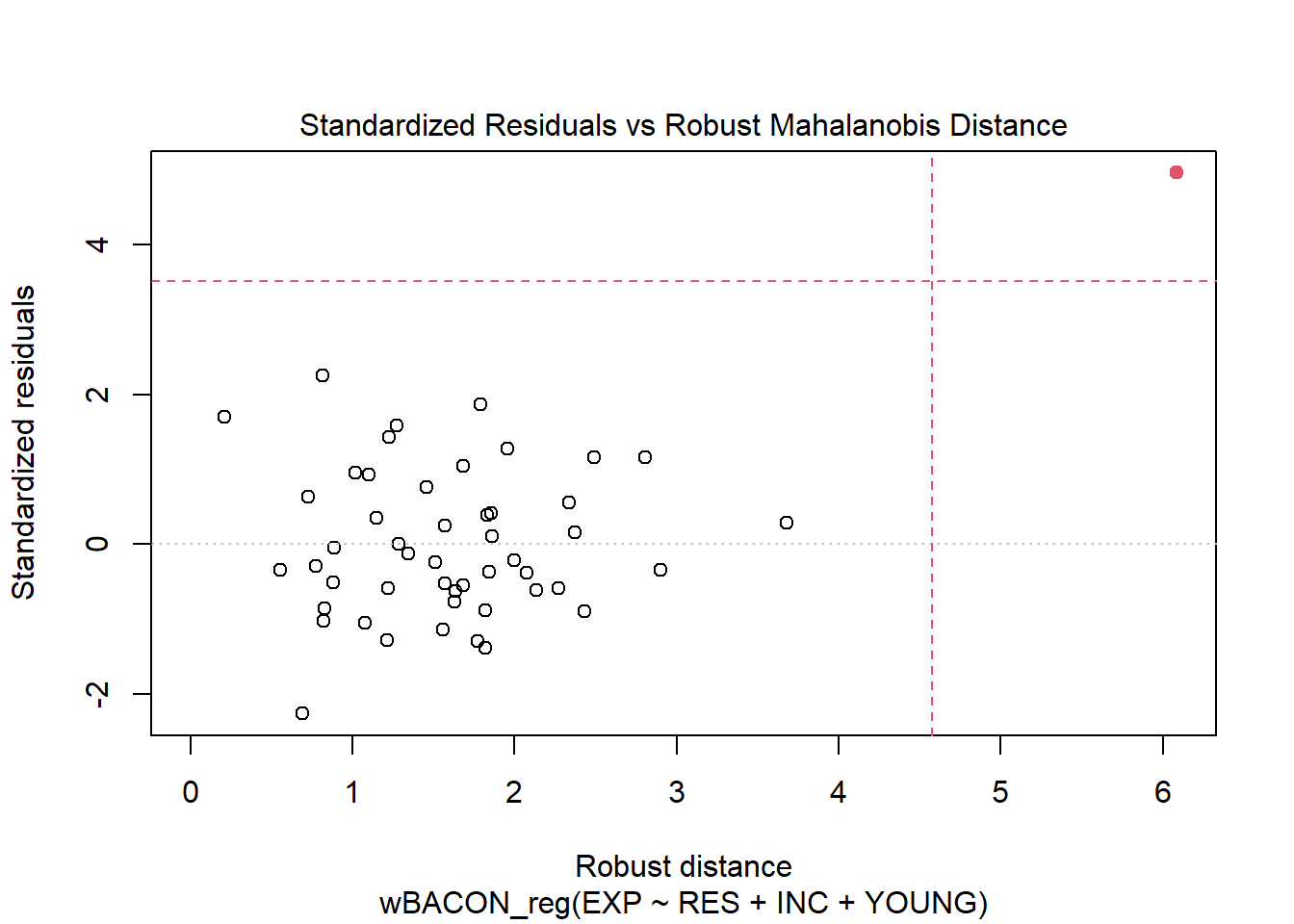## Introduction

The package wbacon implements a weighted variant of the BACON (blocked adaptive computationally-efficient outlier nominators) algorithms Billor et al. (2000) for multivariate outlier detection and robust linear regression. The extension of the BACON algorithm for outlier detection to allow for weighting is due to Béguin and Hulliger (2008).

### Available methods

• wBACON() is for multivariate outlier nomination and robust estimation of location/ center and covariance matrix
• wBACON_reg() is for robust linear regression (the method is robust against outliers in the response variable and the model’s design matrix)

### Assumptions

The BACON algorithms assume that the underlying model is an appropriate description of the non-outlying observations; Billor et al. (2000). More precisely,

• the outlier nomination method assumes that the “good” data have (roughly) an elliptically contoured distribution (this includes the Gaussian distribution as a special case);
• the regression method assumes that the non-outlying (“good”) data are described by a linear (homoscedastic) regression model and that the independent variables (having removed the regression intercept/constant, if there is a constant) follow (roughly) an elliptically contoured distribution.

“Although the algorithms will often do something reasonable even when these assumptions are violated, it is hard to say what the results mean.” Billor et al. (2000, p. 290)

It is strongly recommended that the structure of the data be examined and whether the assumptions made about the “good” observations are reasonable.

### The role of the data analyst

In line with Billor et al. (2000, p. 290), we use the term outlier “nomination” rather than “detection” to highlight that algorithms should not go beyond nominating observations as potential outliers; see also Béguin and Hulliger (2008). It is left to the analyst to finally label outlying observations as such.

The software provides the analyst with tools and measures to study potentially outlying observations. It is strongly recommended to use the tools.

Additional information on the BACON algorithms and the implementation can be found in the documents:

• methods.pdf: A mathematical description of the algorithms and their implementation;
• doc_c_functions.pdf: A documentation of the C functions.

Both documents can be found in the package folder doc.

## Installation

Make sure that the package devtools is installed.1 Then, the wbacon package can be pulled and installed from the GitHub repository using

> devtools::install_github("tobiasschoch/wbacon")

The package contains C code that needs to be compiled. Users of Microsoft Windows need an installation of the R tool chain bundle rtools40 to build the package.

Once the package has been installed, it can be loaded and attached to the current R session by

> library(wbacon)

## Multivariate outlier detection

In this section, we study multivariate outlier detection for the two datasets

• bushfire data (with sampling weights),
• philips data (without sampling weights).

### Bushfire data

The bushfire dataset is on satellite remote sensing. These data were used by Campbell (1984) to locate bushfire scars. The data are radiometer readings from polar-orbiting satellites of the National Oceanic and Atmospheric Administration (NOAA) which have been collected continuously since 1981. The measurements are taken on five frequency bands or channels. In the near infrared band, it is possible to distinguish vegetation types from burned surface. At visible wavelengths, the vegetation spectra are similar to burned surface. The spatial resolution is rather low (1.1 km per pixel).

#### Data preparation

The bushfire data contain radiometer readings for 38 pixels and have been studied in Maronna and Yohai (1995), Béguin and Hulliger (2002), Béguin and Hulliger (2008), and Hulliger and Schoch (2009). The data can be obtained from the R package modi(Hulliger and Sterchi, 2020).2

> data(bushfire, package = "modi")

The first 6 readings on the five frequency bands (variables) are

> head(bushfire)
X1  X2  X3  X4  X5
1 111 145 188 190 260
2 113 147 187 190 259
3 113 150 195 192 259
4 110 147 211 195 262
5 101 136 240 200 266
6  93 125 262 203 271

Béguin and Hulliger (2008) generated a set of sampling weights. The weights can be attached to the current session by

> data(bushfire.weights, package = "modi")

#### Outlier detection

> fit <- wBACON(bushfire, w = bushfire.weights, alpha = 0.05)
> fit

Weighted BACON: Robust location, covariance, and distances
Converged in 3 iterations (alpha = 0.05)
Number of potential outliers: 13 (34.21%)

The argument alpha determines the $$(1-\alpha)$$-quantile $$\chi_{\alpha,d}^2$$ of the chi-square distribution with $$d$$ degrees of freedom.3 All observations whose squared Mahalanobis distances are smaller than the quantile (times a correction factor) are selected into the subset of outlier-free data. It is recommended to choose alpha on grounds of an educated guess of the share of “good” observations in the data. Here, we suppose that 95% of the observations are not outliers.

By default, the initial subset is determined by the Euclidean norm (initialization method: version = "V2").

• This initialization method is robust because it is based on the coordinate-wise (weighted) median. The resulting estimators of center and scatter are not affine equivariant. Let $$T(\cdot)$$ denote an estimator of a parameter of interest (e.g., covariance matrix) and let $$X$$ denote the $$(n \times p)$$ data matrix. An estimator $$T$$ is affine equivariant if and only if $$T(A X + b) = A T(X) + b$$, for any nonsingular $$(m \times n)$$ matrix $$A$$ and any $$n$$-vector $$b$$. Although version "V2" of the BACON method yields an estimator that is not affine equivariant in the above sense, Billor et al. (2000) point out that the method is nearly affine equivariant.
• There exists an alternative initialization method ("version = V1") which is based on the coordinate-wise (weighted) means; therefore, it is affine equivariant but not robust.

From the above output, we see that the algorithm converged in three iterations. In case the algorithm does not converge, we may increase the maximum number of iterations (default: maxiter = 50) and toggle verbose = TRUE to (hopefully) learn more why the method did not converge.

In the next step, we want to study the result in more detail. In particular, we are interested in the estimated center and scatter (or covariance) matrix. To this end, we can call the summary() method on the object fit.

> summary(fit)

Weighted BACON: Robust location, covariance, and distances
Initialized by method: V2
Converged in 3 iterations (alpha = 0.05)

Number of potential outliers: 13 (34.21%)

Robust estimate of location:
X1    X2    X3    X4    X5
108.4 149.0 275.6 218.7 279.8

Robust estimate of covariance:
X1     X2      X3     X4     X5
X1   397.3  301.4 -1368.2 -268.1 -227.0
X2   301.4  258.9  -916.2 -161.3 -143.2
X3 -1368.2 -916.2  7262.8 1757.8 1406.6
X4  -268.1 -161.3  1757.8  472.2  368.5
X5  -227.0 -143.2  1406.6  368.5  290.4

Distances (cutoff: 5.675):
Min. 1st Qu.  Median    Mean 3rd Qu.    Max.
1.321   1.923   2.534   6.957  13.082  20.550 

#### Diagnostics

The method has detected 13 potential outliers. It is important to study the diagnostic plot to learn more about the potential outliers. The robust (Mahalanobis) distances vs. the index of the observations (1:n) can be plotted as follows.

> plot(fit, 1)The dashed horizontal line shows the cutoff threshold on the robust distances. Observations above the line are nominated as potential outliers by the BACON algorithm. It is left to the analyst to finally label outlying observations as such. In the next section, we introduce an alternative plotting method (see below).

The method is_outlier() returns a vector of logicals whether an observation has been flagged as a potential outlier.

> which(is_outlier(fit))
  7  8  9 10 11 12 32 33 34 35 36 37 38

The (robust) center and covariance (scatter) matrix can be extracted with the auxiliary functions, respectively, center() and cov().

> center(fit)
X1       X2       X3       X4       X5
108.4462 148.9538 275.5692 218.6769 279.7538 

The robust Mahalanobis distances can be extracted with the distance() method.

### Philips data

Old television sets had a cathode ray tube with an electron gun. The emitted beam runs through a diaphragm that lets pass only a partial beam to the screen. The diaphragm consists of 9 components. The Philips data set contains $$n = 667$$ measurements on the $$p = 9$$ components (variables); see Rousseeuw and van Driessen (1999).

The philips data can be loaded from the R package cellWise (Raymaekers and Rousseeuw, 2020). These data do not have sampling weights.

> data(data_philips, package = "cellWise")
X1     X2    X3    X4    X5    X6     X7     X8     X9
[1,] 0.153 -0.259 0.140 0.514 2.242 0.443 -0.021 -0.035 -0.065
[2,] 0.119 -0.309 0.132 0.518 2.269 0.458 -0.018 -0.035 -0.053
[3,] 0.173 -0.296 0.138 0.516 2.266 0.461 -0.023 -0.026 -0.052
[4,] 0.135 -0.306 0.139 0.522 2.288 0.464 -0.015 -0.031 -0.051
[5,] 0.143 -0.278 0.139 0.519 2.284 0.465 -0.016 -0.018 -0.054
[6,] 0.140 -0.284 0.159 0.531 2.287 0.465 -0.004 -0.024 -0.052

We compute the BACON algorithm but this time with the initialization method version = "V1".

> fit <- wBACON(data_philips, alpha = 0.05, version = "V1")
> fit

Weighted BACON: Robust location, covariance, and distances
Converged in 7 iterations (alpha = 0.05)
Number of potential outliers: 82 (12.11%)

The BACON algorithm detected 82 potential outliers. The robust (Mahalanobis) distances can be plotted against the univariate projection of the data, which maximizes the separation criterion of Qiu and Joe (2006). This kind of diagnostic graph attempts to separate outlying from non-outlying observations as much as possible; see Willems et al. (2009). It is helpful if the outliers are clustered. The graph is generated as follows.

> plot(fit, which = 2)From the visual display, we see a cluster of potential outliers in the top right corner. The dashed horizontal line indicates the cutoff threshold on the distances as imposed by the BACON algorithm.

For very large datasets, the plot method can be called with the (additional) argument hex = TRUE to show a hexagonally binned scatterplot; see below. This plot method uses the functionality of the R package hexbin (Carr et al., 2021).

> plot(fit, which = 2, hex = TRUE)## Robust linear regression

The education data is on education expenditures in 50 US states in 1975 (Chatterjee and Hadi, 2012, Chap. 5.7). The data can be loaded from the robustbase package.

> data(education, package = "robustbase")

It is convenient to rename the variables.

> names(education)[3:6] <- c("RES", "INC", "YOUNG", "EXP")
State Region RES  INC YOUNG EXP
1    ME      1 508 3944   325 235
2    NH      1 564 4578   323 231
3    VT      1 322 4011   328 270
4    MA      1 846 5233   305 261
5    RI      1 871 4780   303 300
6    CT      1 774 5889   307 317

The measured variables for the 50 states are:

• State: State
• Region: group variable with outcomes: 1=Northeastern, 2=North central, 3=Southern, and 4=Western
• RES: Number of residents per thousand residing in urban areas in 1970
• INC: Per capita personal income in 1973 ($US) • YOUNG: Number of residents per thousand under 18 years of age in 1974 • EXP: Per capita expenditure on public education in a state ($US), projected for 1975

### Model fit

We want to regress education expenditures (EXP) on the variables RES, INC, and YOUNG by the BACON algorithm, and obtain

> reg <- wBACON_reg(EXP ~ RES + INC + YOUNG, data = education)
> reg

Call:
wBACON_reg(formula = EXP ~ RES + INC + YOUNG, data = education)

Regression on the subset of 49 out of 50 observations (98%)

Coefficients:
(Intercept)          RES          INC        YOUNG
-277.57731      0.06679      0.04829      0.88693  

The instance reg is an object of the class wbaconlm. The printed output of wBACON_reg is identical with the one of the lm function. In addition, we are told the size of the subset on which the regression has been computed. The observations not in the subset are considered outliers (here 1 out of 50 observations).

The summary() method can be used to obtain a summary of the estimated model.

> summary(reg)

Call:
wBACON_reg(formula = EXP ~ RES + INC + YOUNG, data = education)

Residuals:
Min      1Q  Median      3Q     Max
-81.128 -22.154  -7.542  22.542  80.890

Coefficients:
Estimate Std. Error t value Pr(>|t|)
(Intercept) -277.57731  132.42286  -2.096 0.041724 *
RES            0.06679    0.04934   1.354 0.182591
INC            0.04829    0.01215   3.976 0.000252 ***
YOUNG          0.88693    0.33114   2.678 0.010291 *
---
Signif. codes:  0 '***' 0.001 '**' 0.01 '*' 0.05 '.' 0.1 ' ' 1

Residual standard error: 35.81 on 45 degrees of freedom
Multiple R-squared:  0.4967,    Adjusted R-squared:  0.4631
F-statistic:  14.8 on 3 and 45 DF,  p-value: 7.653e-07

The summary output of wBACON_reg is identical with the output of the lm estimate on the subset of outlier-free data,

> summary(lm(EXP ~ RES + INC + YOUNG, data = education[!is_outlier(reg), ]))

Call:
lm(formula = EXP ~ RES + INC + YOUNG, data = education[!is_outlier(reg),
])

Residuals:
Min      1Q  Median      3Q     Max
-81.128 -22.154  -7.542  22.542  80.890

Coefficients:
Estimate Std. Error t value Pr(>|t|)
(Intercept) -277.57731  132.42286  -2.096 0.041724 *
RES            0.06679    0.04934   1.354 0.182591
INC            0.04829    0.01215   3.976 0.000252 ***
YOUNG          0.88693    0.33114   2.678 0.010291 *
---
Signif. codes:  0 '***' 0.001 '**' 0.01 '*' 0.05 '.' 0.1 ' ' 1

Residual standard error: 35.81 on 45 degrees of freedom
Multiple R-squared:  0.4967,    Adjusted R-squared:  0.4631
F-statistic:  14.8 on 3 and 45 DF,  p-value: 7.653e-07

where we have used is_outlier() to extract the set of declared outliers from reg (the summary output of the lm estimate is not shown).

### Tuning

By default, wBACON_reg uses the parametrization $$\alpha = 0.05$$, collect = 4, and version = "V2". These parameters are used to call the wBACON algorithm on the design matrix. Then, the same parameters are used to compute the robust regression.

To ensure a high breakdown point, version = "V2" should not be changed to version = "V1" unless you have good reasons. The main “turning knob” to tune the algorithm is alpha, which defines the $$(1-$$alpha$$)$$ quantile of the Student $$t$$-distribution. All observations whose distances/discrepancies [See document methods.pdf in the folder doc of the package.] are smaller (in absolute value) than the quantile are selected into the subset of “good” data. By choosing smaller values for alpha (e.g., 0.2), more observations are selected (ceteris paribus) into the subset of “good” data (and vice versa).

The parameter collect specifies the initial subset size, which is defined as $$m = p \cdot collect$$. It can be modified but should be chosen such that $$m$$ is considerably smaller than the number of observations $$n$$. Otherwise there is a high risk of selecting too many “bad” observations into the initial subset, which will eventually bias the regression estimates.

In case the algorithm does not converge, we may increase the maximum number of iterations (default: maxiter = 50) and toggle verbose = TRUE to (hopefully) learn more why the method did not converge.

### Model diagnostics

The methods coef(), vcov(), and predict() work exactly the same as their lm counterparts. This is also true for the first three plot types, that is

• which = 1: Residuals vs Fitted,
• which = 2: Normal Q-Q,
• which = 3: Scale-Location

The plot types 4:6 of plot.lm are not implemented for objects of the class wbaconlm because it is not sensible to study the standard regression influence diagnostics in the presence of outliers in the model’s design space. Instead, type four (which = 4) plots the robust Mahalanobis distances with respect to the non-constant design variables against the standardized residual. This plot has been proposed by Rousseeuw and van Zomeren (1990).

> plot(reg, 4)The filled circle(s) represent the outliers nominated by the BACON algorithm. The outlier in the top right corner is both a residual outlier and an outlier in the model’s design space.

• Observation with robust Mahalanobis distances larger than 4.57 (see abscissae) are flagged as outliers in the model’s design space (leverage observations).
• Observations whose standardized residual falls outside the interval spanned by $$\pm \, t_{\alpha/(2m+2), m - p}$$, where $$t_{\alpha, m - p}$$ is the $$(1-\alpha)$$ quantile of the Student $$t$$-distribution with $$m-p$$ degrees of freedom, $$m$$ denoting the size of the final subset of outlier-free data. Here, we have $$m=49$$, $$\alpha = 0.05$$ (see argument alpha of wBACON_reg), thus the interval is $$[-3.52, \; 3.52]$$.

## References

Béguin, C. and B. Hulliger (2002). Robust Multivariate Outlier Detection and Imputation with Incomplete Survey Data, Deliverable D4/5.2.1/2 Part C: EUREDIT project, https://www.cs.york.ac.uk/euredit/euredit-main.html, research project funded by the European Commission, IST-1999-10226.

Béguin, C. and B. Hulliger (2008). The BACON-EEM Algorithm for Multivariate Outlier Detection in Incomplete Survey Data, Survey Methodology, Vol. 34, No. 1, 91–103.

Billor, N., A. S. Hadi, and P. F. Vellemann (2000). BACON: Blocked Adaptive Computationally-efficient Outlier Nominators, Computational Statistics and Data Analysis, 34, 279–298.

Campbell, N. A. (1989). Bushfire Mapping using NOAA AVHRR Data. Technical Report. Commonwealth Scientific and Industrial Research Organisation, North Ryde.

Carr, D., N. Lewin-Koh, and M. Maechler (2021). hexbin: Hexagonal Binning Routines. R package version 1.28.2. (The package contains copies of lattice functions written by Deepayan Sarkar).

Chatterjee, S. and A. H. Hadi (2012). Regression Analysis by Example, 5th ed., Hoboken (NJ): John Wiley & Sons.

Hulliger, B. and T. Schoch (2009). Robust multivariate imputation with survey data, in Proceedings of the 57th Session of the International Statistical Institute, Durban.

Hulliger, B. and M. Sterchi (2020). modi: Multivariate Outlier Detection and Imputation for Incomplete Survey Data, R package version 0.1-0.

Maechler, M., P. Rousseeuw, C. Croux, V. Todorov, A. Ruckstuhl, M. Salibian-Barrera, T. Verbeke, M. Koller, E. L. T. Conceicao, and M. Anna di Palma (2020). robustbase: Basic Robust Statistics, R package version 0.93-6.

Maronna, R. A. and V. J. Yohai (1995). The Behavior of the Stahel-Donoho Robust Multivariate Estimator, Journal of the American Statistical Association, 90, 330–341.

Qiu, W. and H. Joe (2006). Separation index and partial membership for clustering, Computational Statistics and Data Analysis 50, pp. 585-603.

Raymaekers, J. and P. Rousseeuw (2020). cellWise: Analyzing Data with Cellwise Outliers, R package version 2.2.1.

Rousseeuw, P. J. and K. van Driessen (1999). A fast algorithm for the Minimum Covariance Determinant estimator, Technometrics, 41, 212–223.

Rousseeuw, P. J. and K. van Zomeren (1990). Unmasking Multivariate Outliers and Leverage Points, Journal of the American Statistical Association, 411, 633–639.

Willems, G., H. Joe, and R. Zamar (2009). Diagnosing Multivariate Outliers Detected by Robust Estimators, Journal of Computational and Graphical Statistics 18, pp. 73-91.